# Test: Slope & Deflection Level - 2

## 15 Questions MCQ Test Engineering Mechanics | Test: Slope & Deflection Level - 2

Description
Attempt Test: Slope & Deflection Level - 2 | 15 questions in 40 minutes | Mock test for Mechanical Engineering preparation | Free important questions MCQ to study Engineering Mechanics for Mechanical Engineering Exam | Download free PDF with solutions
QUESTION: 1

### For the beam shown in the figure, the elastic curve between the supports B and C will be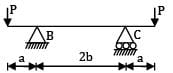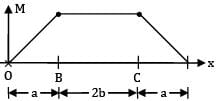Between B and C, BMD is a straight line BM = Constant

Now,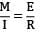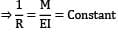⇒ R = Constant Means curve of constant radius.

Hence, circular.

QUESTION: 2

### The maximum deflection of a cantilever of 10.0 m span, an EI = 200.0MN/m2 subjected to a distributed load of 8.0 kN/m is

Solution: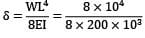= 0.05m = 50mm

QUESTION: 3

### A cantilever of length (L) carries a distributed load whose intensity varies uniformly from zero at the fixed end to ‘w’ per unit length at the free end. The deflection at the free end will be

Solution: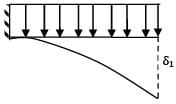δ1 =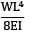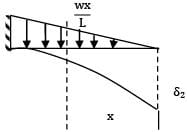δ2 =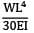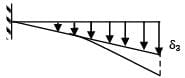δ3 = δ1 − δ2 =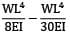=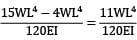QUESTION: 4

If the deflection at the free end of a uniformly loaded cantilever beam is 15 mm and the slope of the deflection curve at the free end is 0.02 radian. then the length of the beam is

Solution: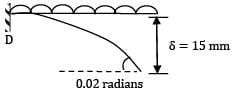Deflection at free end, δ =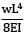= 15 mm

Slope at free end, θ =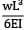= 0.02

∴ From the two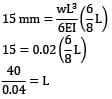L = 1 m

QUESTION: 5

A cantilever beam of uniform cross-section and length L is subjected to upward force W at mid-span and downward force P at the free end. For the deflection to be zero at the free end. W and P are related by

Solution: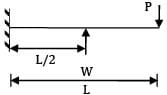Deflection at free end due to load, p =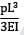Deflection at free end due to support load W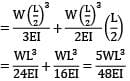∴ The deflection at free end is zero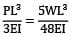W = 16/5P

QUESTION: 6

The slope at the free end of a cantilever of length 1 m is 1°. If the cantilever carries uniformly distributed load over the whole length then the deflection at the free end will be

Solution: δ =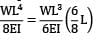=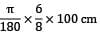= 1.309cm

QUESTION: 7

A simply supported beam 'A' of length L carries a central point load W. Another beam 'B' is loaded with a uniformly distributed load such that the total load on the beam is W. The ratio of maximum deflections between the beams A and B is

Solution: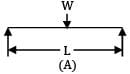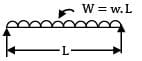Maximum deflection of beam A,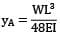Maximum deflection of beam B,

yB =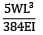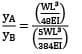=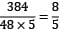QUESTION: 8

A simply supported beam of span 4m subjected to a point load of 4 kN at a distance of 3m from the left support. The maximum slope occurs

Solution: The load is closer to right support; the slope is max at right.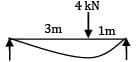QUESTION: 9

If the depth of a simply supported rectangular beam subjected to concentric load at the Centre is doubled, the deflection at the Centre will be reduced to

Solution: y1 =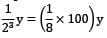= 12.5%
QUESTION: 10

A simply supported rectangular beam of span L and depth d carries a central load W, modulus of elasticity is E, the ratio of maximum deflection to maximum bending stress is

Solution: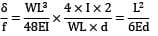QUESTION: 11

A cantilever of span L subjected to uniformly distributed gravity loading w/m is subjected to an upward force P at a distance L/2 from the free end. If the deflection at the free end of the beam is zero, (wL/P) is

Solution: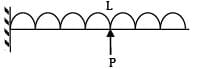Deflection at free end due to vertical load

δ1 =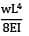Deflection at free end due to support P

δ2 =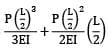=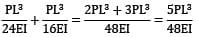∴ Deflection at free end is zero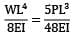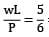= 0.83

QUESTION: 12

A simply supported beam of 10m span is carrying a load of 4.8kN at mid-span. If Young's modulus of elasticity (E) is 2 x 108 kN/m2 and moment of inertia (I) is 20 cm4 , then the maximum deflection will be

Solution:

Maximum deflection (y) =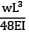=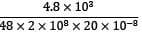= 2.5mm

QUESTION: 13

Two cantilever beams A and B are shown in the figure. The ratio of maximum deflections of beam A to the beam B is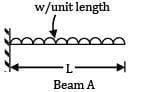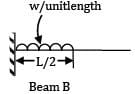Solution: δA =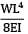δB =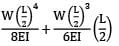=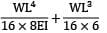=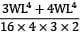=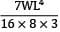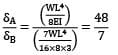QUESTION: 14

A loaded cantilever beam is shown below. The maximum deflection in the beam is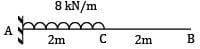Solution: δ =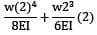=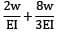=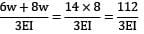QUESTION: 15

A cantilever of length ‘L’ carries a uniformly distributed load of ‘w’ per unit of span over the outer half. The maximum deflection is

Solution: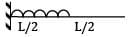ymax =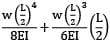=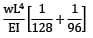ymax =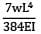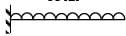ymax =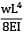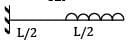ymax =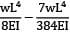=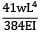Use Code STAYHOME200 and get INR 200 additional OFF Use Coupon Code